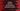# Python program to find the first odd Abundant or excessive number## Python program to find the first odd Abundant or excessive number :

In this tutorial, we will learn how to find the_ first odd Abundant number_ in python. A number is called Abundant or Excessive number if its sum of all proper divisors is more than the number itself. A proper divisor is any divisor of a number other than the number itself. For a prime number, the only proper divisor is ‘1’. ‘1’ doesn’t have any proper divisor. 6 has two proper divisors 2 and 3.

12 is an abundant number. Its proper divisors are 2, 3, 4, and 6. The sum of all proper divisors is 2+ 3+ 4+ 6 = 15, which is greater than 12.

14 is not an abundant number. Its proper divisors are 2 and 7. The sum is 2 + 7 =9, which is less than 14.

List of all abundant numbers from 1 to 100 are 12, 18, 20, 24, 30, 36, 40, 42, 48, 54, 56, 60, 66, 70, 72, 78, 80, 84, 88, 90, 96 and 100. Have you noticed all are even here ? In this tutorial, we will learn how to find the first odd Abundant number using Python.

We will use one infinite while loop that will check for all numbers starting from 1. If any number is abundant and also odd, it will print out the result.

### Python 3 program :

``````#5
def isAbundant(input_no):
#6
total = 0

#7
for i in range(1,input_no):
#8
if(input_no % i == 0):
#9
total = total + i
if(total > input_no):
return True

#10
if(total > input_no):
return True
else :
return False

#1
no = 1
while(True):
#2
if(isAbundant(no) and no%2 != 0):
#3
print("Odd abundant no : ",no)
break
#4
no += 1``````

The source code is available here.

## Explanation :

The commented numbers in the above program denote the step numbers below :

1. Initialize one variable no to 1 and run one infinite loop. We are using while loop as infinite. This will check for all numbers starting from 1. For each number, it will check if it is abundant or not.
2. Check if the current number is abundant or not. Also, check if it is odd or not. For checking abundant, we are calling the isAbundant method and for checking even or not, we are using modulo operator. Both conditions are combined using ‘and’ operator.
3. If it is odd and abundant, print out the number and break from the infinite loop. It will exit from the infinite loop and stop the program.
4. Increase the no in the while loop so that we can check the next number. The while loop is checking each number one by one.
5. isAbundant function takes one number as a parameter and returns True if a number is Abundant. Else, it returns False.
6. Set value of total as 0. total variable is used to hold the sum of all proper divisors for a number.
7. Run one for loop to run from 1 to the input number. This loop is used to find out the proper divisors for a number.
8. Check if the number is divisible by the current for loop index or check if the current index of the loop is a proper divisor for the number or not.
9. If it is a proper divisor, add this value to the total. If the total becomes more than the input number, return True.
10. Finally, check if the total sum is more than the input number or not. If yes, return True, else return False.

### Output :

```````Odd abundant no :  945
```````

So, the first abundant number is 945.

### Conclusion :

In this tutorial, we have learned how to find out the first odd abundant or excessive number in python. Using the same program, you can print out the first ‘n’ abundant numbers. Try to run the program and drop one comment below if you have any queries.